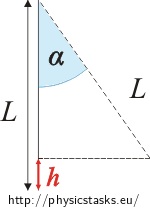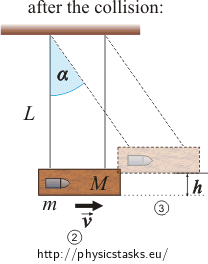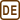## Ballistic pendulum 1

A bullet with mass m hits a ballistic pendulum with length L and mass M and lodges in it. When the bullet hits the pendulum it swings up from the equilibrium position and reaches an angle α at its maximum. Determine the bullet’s velocity.

Note: The ballistic pendulum was used for measuring the speed of a bullet. It consists of a wooden block suspended from two long cords so that it can swing only in the vertical direction.

• #### Hint 1 - deflection of the pendulum

How high does the pendulum hit by the bullet rise? Draw yourself a figure.

• #### Hint 2 - momentum

Because the bullet lodges in the pendulum’s body, we can say that the collision was inelastic. What can one say about the momentum before and after the collision?

• #### Hint 3 - energy

During a perfectly inelastic collision part of the kinetic energy converts into internal energies of the bullet and the pendulum. Thus, we can not use the law of conservation of energy in comparing the situations before (1) and after (2) the collision. After the bullet has lodged in the pendulum, the energy is conserved (neglecting air resistance). Compare energies at the moment immediately before the collision (2) and at the moment the pendulum reaches the maximum deflection (3).

The initial velocity of the bullet before the collision is $$v_1=\sqrt {2gL(1-\cos \alpha)}(1+\frac {M}{m})$$.

• #### Complete solution

At first we express the maximum height to which the pendulum rised.$$L$$ ... the length of the pendulum

$$\alpha$$ ... the angle of the pendulum’s deflection

$$h$$ ... the vertical height through which the pendulum rises

Express the dependence of the height h, through which the pendulum rises, on the pendulum’s length and on the angle of deflection:

$h=L-L\cos \alpha=L(1-\cos \alpha)\tag{1}$

Than we use the laws of conservation of energy and of linear momentum.$$m$$ ... the mass of the bullet

$$M$$ ... the mass of the pendulum

$$v_1$$ ... the initial velocity of the bullet

$$v_2$$ ... the initial velocity of the pendulum

$$v$$ ... the velocity of the pendulum-bullet system immediately after the collision

The law of conservation of momentum states that in an isolated system the total momentum is constant. This law works also during perfectly inelastic collision.

$\vec{p_1}+\vec{p_2}=\vec{p}$

$$\vec{p_1}$$ ... the momentum vector of the bullet before the collision

$$\vec{p_2}$$ ... the momentum vector of the pendulum before the collision

$$\vec{p}$$ ... the momentum vector of the pendulum-bullet system immediately after the collision

$m\vec{v_1}+M\vec{v_2}=(m+M)\vec{v}$

The pendulum is at rest before the collision, $$v_2 = 0$$:

$m\vec{v_1}=(m+M)\vec{v}$

Vectors $$\vec{v_1}$$ and $$\vec{v }$$ are collinear and so we can rewrite the equation with scalar values (without vectors):

$mv_1=(m+M)v$ $v=\frac{mv_1}{m+M}\tag{2}$

During a perfectly inelastic collision part of the kinetic energy converts into internal energies of the bullet and the pendulum. Thus, we can not use the law of conservation of energy in comparing the situations before (1) and after (2) the collision. After the bullet has lodged in the pendulum, the energy is conserved (neglecting air resistance). Compare energies at the moment immediately before the collision (2) and at the moment the pendulum reaches the maximum deflection (3).

The law of conservation of mechanical energy:

The total amount of mechanical energy in a closed system remains constant over time, or the sum of potential energy and kinetic energy is constant.

The zero level of potential energy is chosen in the situation 2.

$E_k=E_p$

$$E_k$$ ... kinetic energy of the bullet-pendulum system immediately after collision

$$E_p$$ ... potential energy of the bullet-pendulum system at the moment the pendulum reaches the maximum deflection

$\frac{1}{2}(m+M)v^2=(m+M)gh\tag{3}$

Insert the velocity v from equetion (2):

$\frac{1}{2}(m+M)\frac{m^2v_1^2}{(m+M)^2}=(m+M)gh$

We next express the desired initial velocity of the bullet v1 in terms of the other variables:

$v_1=\sqrt {2gh}(1+\frac{M}{m})$

Insert the height h from equation (1):

$v_1=\sqrt {2gL(1-\cos \alpha )}(1+\frac{M}{m})$

Note:

The law of conservation of energy for situations before and after the collision could be written as:

$E_{ks}=E_k+\Delta U$

$$E_{ks}$$ ... kinetic energy of the bullet before the collision

$$E_k$$ ... kinetic energy of the bullet-pendulum system immediately after the collision

$$\Delta U$$ ... the change in internal energy

$\frac{1}{2}mv_1^2=\frac{1}{2}(m+M)v^2+\Delta U$